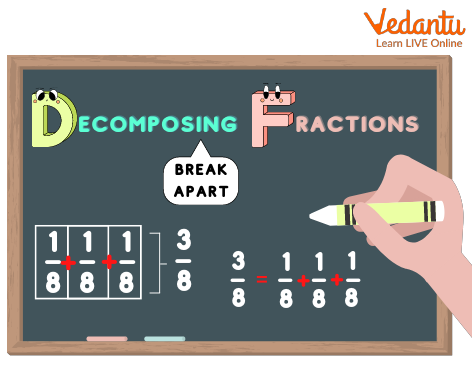Courses
Courses for Kids
Free study material
Free LIVE classes
More

# Decomposing of FractionsLIVE
Join Vedantu’s FREE Mastercalss

You must be familiar with the concept of fractions; in this section, we will learn how fractions are split up or divided into smaller fractions forming decomposed fractions.

We will be learning the methods of decomposing fractions and mixed fractions. This study is done with the help of several examples so that the students understand the concept of decomposing fractions in an effective manner.

## What Is Meant by Decomposing Fractions?Decomposition of fractions

In real terms, the meaning of decompose means ‘splitting up’ or ‘dividing the fractions into smaller parts or chunks. When we decompose a fraction, we divide it into smaller fractions. It is to be noted that the decomposed fractions or the smaller fractions add up to form the initial fraction.

### How Can You Decompose Fractions?

We can decompose fractions by breaking them into unit fractions.

What Are Unit Fractions?

Unit fractions are those fractions in which the numerator is 1. For example - ⅓, ⅕, etc. This means that a unit fraction is a part of a whole unit or a part of 1.

### Larger Fractions into Many Unit Fractions

The easiest way to break larger fractions is by decomposing them into many unit fractions. Like ⅝ is the same as ⅛, ⅛, ⅛, ⅛, ⅛, which is fives times ⅛. Let us take another example. Consider the fraction ⅚; this means there are 5 parts among 6 parts in total. This can be decomposed as ⅙, ⅙, ⅙, ⅙, ⅙.

### Sum of Smaller Fractions That Are Not Unit Fractions

There is yet another way to decompose larger fractions. We can decompose a large fraction into smaller fractions. This method of decomposition of large fractions into smaller ones can be applied where we cannot decompose the larger fraction into unit fractions, as observed in the previous section.

We can also decompose a fraction by using the sum of smaller fractions.

Like - ⅚ can also be split into 1⁄6, 1⁄6, and 3⁄6 or 2⁄6 and 3⁄6 or 1⁄6 and 4⁄6

Another example - 5⁄6 = 2⁄6 + 3⁄6 = 1⁄3 + 1⁄2

Here, we can again simplify the fraction as 2⁄6 = 1⁄3 and 3⁄6 = 1⁄2

### Decomposing Mixed Fractions

A mixed fraction is a combination of a whole number and a proper fraction, which is represented together. The mixed fraction represents a number that is between any two of the whole numbers.

The numerator and denominator in the mixed fraction are a part of the proper fraction, thereby forming the mixed number. The result, after we split a mixed fraction, is a whole number and a proper fraction.

### Conclusion

One can study the decomposition of fractions only with a proper understanding of fractions. For parents, in order to help their kids, it's always advisable to use images and other visual representations which will help them to grasp the method of decomposition.

Also, another note to remember, while working with fractions, you can only add or subtract the parts which refer to the same size or the whole.

Last updated date: 19th Sep 2023
Total views: 136.8k
Views today: 2.36k

## FAQs on Decomposing of Fractions

1. What does decompose mean in the study of Mathematics?

In the study of Mathematics, decomposing means breaking the whole number into smaller parts.

2. What do you mean by partial fractions?

Partial fractions are fractions that were used in the process of decomposing rational fractions. When an algebraic expression is being divided into a sum of two or more rational numbers, each of the parts is called a partial fraction.